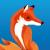## perl日志分析，求平均值

songoex 发布于 2016/10/19 22:04

numbs:1234 start

something in english

numbs:1256 end

something in english

..........................

0```\$start=\$end=undef;
\$cnt=\$sum=0;
open DATAFILE,'datafile.dat';
while (<DATAFILE>){

\$start=\$1 if /numbs:(\d+) start/;
\$end=\$1 if /numbs:(\d+) end/;

if(\$start && \$end){

\$cnt++;
\$sum+=\$end-\$start;
\$start=\$end=undef;

}
}
print \$sum/\$cnt;```